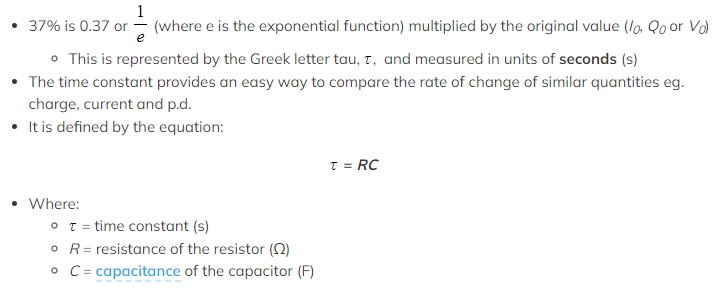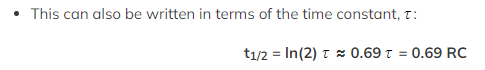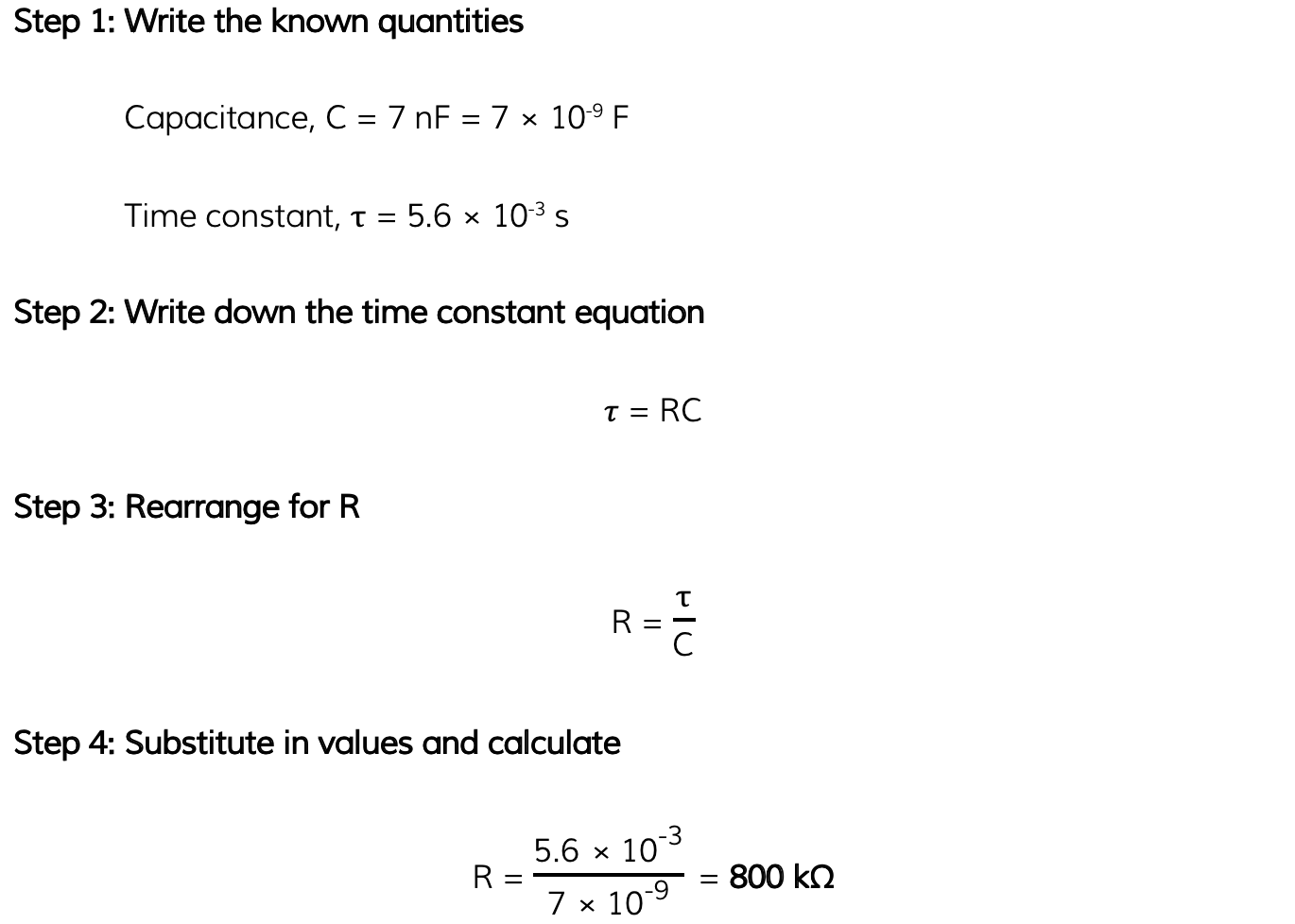# IB DP Physics: HL复习笔记11.3.4 The Time Constant

### The Time Constant

• The time constant of a capacitor discharging through a resistor is a measure of how long it takes for the capacitor to discharge
• The definition of the time constant is:

The time taken for the charge, current or voltage of a discharging capacitor to decrease to 37% of its original value

• Alternatively, for a charging capacitor:

The time taken for the charge or voltage of a charging capacitor to rise to 63% of its maximum value

•The graph of voltage-time for a discharging capacitor showing the positions of the first three time constants

• The time to half, t1/2 (half-life) for a discharging capacitor is:

The time taken for the charge, current or voltage of a discharging capacitor to reach half of its initial valueExam Tip

Note that the time constant is not the same as half-life. Half-life is how long it takes for the current, charge or voltage to halve whilst the time constant is to 37% of its original value (not 50%).

Although the time constant is given on the datasheet, you will be expected to remember the half-life equation t1/2 = 0.69RC

### Problems Involving the Time Constant

• Problems involving the time constant tend to involve
• Calculations
• Determining the time constant from a graph
• To find the time constant from a voltage-time graph, calculate 0.37V0 and determine the corresponding time for that valueThe time constant shown on a charging and discharging capacitor

#### Worked Example

A capacitor of 7 nF is discharged through a resistor of resistance R. The time constant of the discharge is 5.6 × 10-3 s.

Calculate the value of R.